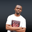Related Tags

math
euphoria
functions
communitycreator

# How to calculate the square root in EuphoriaNDUKWE CHIDERA K.

### Overview

To calculate the square root of any value in Euphoria, we leverage the math method, sqrt().

### The sqrt() method

The sqrt() method in Euphoria is part of the standard math library and returns the square root of any integer it is provided with.

### Syntax

sqrt(value)


### Parameter

value: This is an integer or sequence of integer values whose square root value is to be computed.

### Return Value

The sqrt() method returns the square root of the number or the sequence of numbers provided.

### Code

To run the code below or any code using math methods, we have to include the std/math.e file in such a program. We can see this here:

include std/math.e

--declare variables
sequence seq1, seq2

--assign values to sequence declared
seq1 = {4,16,36,49,64}
seq2 = {3,5,7,9,15}

--print the values of some numbers

print(1, sqrt(144))
--get square root of seq1 and seq2
puts(1,"\nThe square root of {4,16,36,49,64} is: ")
print(1,sqrt(seq1))

puts(1,"\nThe square root of {3,5,7,9,15} is: ")
print(1,sqrt(seq2))
Simple example using the sqrt() method

### Explanation

• Line 1: We include the math module into code.
• Line 4: We declare some variables seq1 and seq2.
• Line 7 and Line 8: We assign values to the declared variables.
• Line 12: We print the square root of 144 to the screen.
• Line 15 and 18: We calculate and print the square roots of seq1 and seq2.

RELATED TAGS

math
euphoria
functions
communitycreator

CONTRIBUTORNDUKWE CHIDERA K.
RELATED COURSES

View all Courses

Keep Exploring

Learn in-demand tech skills in half the time